Home Beams Analysis of Continuous Beams with Partially Distributed Load Using Force Method and...

# Analysis of Continuous Beams with Partially Distributed Load Using Force Method and Clapeyron’s Theorem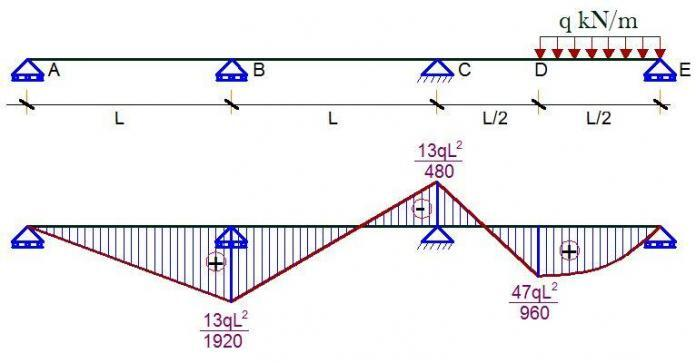For the continuous beam loaded as shown above, it is desired to find the bending moments at the critical points using force method (method of consistent deformations) and Clapeyron’s theorem (3 Moment Equation). We should however note that both methods are force methods (flexibility method) since we generally solve for unknown forces.

Solution

(1) By force method
Degree of static indeterminacy neglecting horizontal forces and reactions.

D = 2m + r – 2n
D = 2(3) + 4 – 2(4) = 2
Therefore the structure is indeterminate to the 2nd order.

Basic System
A basic system is a system that is statically determinate and stable. This obtained by removing the redundant supports at A and B, and replacing them with unit loads. See the figure below.Case 1

Bending moment on the basic system due to vertical unit virtual load at support A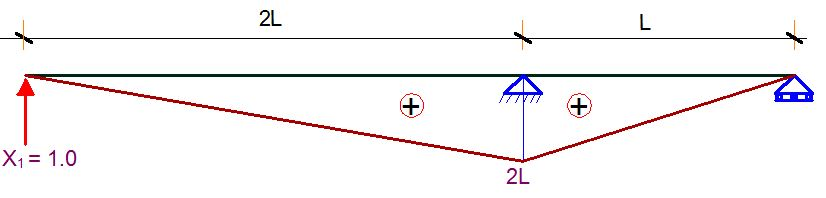Case 2
Bending moment on the basic system due to unit virtual load at support BCase 3
Bending moment due to externally applied load on the basic system;Influence Co-efficients

δ11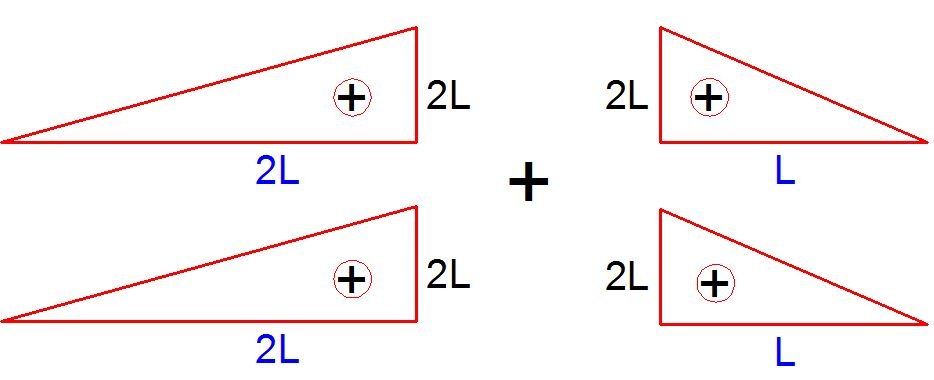δ11 = [1/3 × 2L × 2L × 2L] + [1/3 × 2L × 2L × L] = 4L3

δ22δ11 = 2[1/3 × L × L × L] = 2L3/3

δ21 = δ12δ11 = [1/6 × L × L(4L + L)] + [1/3 × L × L × L] = 3L3/2

δ1P

δ1P = [1/6 × qL2/16 × (2L + 2L) × L/2]] + [15/12 × qL2/16 × L × L/2] = 13qL4/384

δ2P

δ2P = [1/6 × qL2/16 × (L + L) × L/2] + [15/12 × qL2/16 × L/2× L/2] = 13qL4/768

The appropriate cannonical equation is given by;

δ11X1 +  δ12X2 + δ1P = 0
δ21X1 +  δ22X2 + δ2P = 0

Therefore;

(4L3)X1 +  (3L3/2)X2  = -13qL4/384
(3L3/2)X1 +  (2L3/3)X2  = -13qL4/768

On sloving the above equations simultaneously;

X1 = 13qL/1920 KN

X2 = -13qL/320 KN

The final moment values can now be computed;

Mf = M1X1 + M2X2 + MP

MB = (13qL/1920 × L) + 0 + 0 = 13qL2/1920
MC = (13qL/1920 × 2L) – (13qL/230 × L) + 0 = -13qL2/480
MD = (13qL/1920 × L) – (13qL/230 × L/2) + qL2/16  = 47qL2/960

(2) By Clapeyron’s Theorem;

First of all, we draw the free bending moment diagram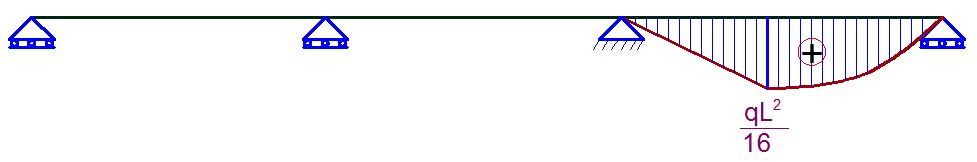By Clapeyron’s three moment equation (EI = constant, no sinking of support);

MAL1 + 2MB + MCL2 + 6A1X1 + 6A2X= 0

Geometrical Properties of the free moment diagram (centroid)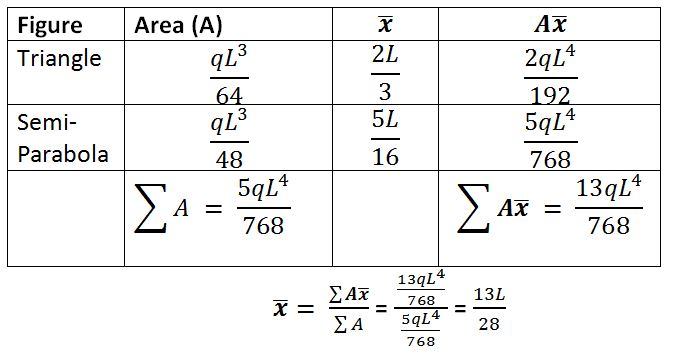SPAN A – C
MA = 0
2MB(L + L) + MCL = 0
4MB L + MCL = 0 ——————– (1)

SPAN B-D
MD = 0
MBL + 2MC(L + L) = [-6 × 7qL3/192 × 13L/128]/L
MB L + 4MCL =  -13qL3/128 ——————– (2)

Solving (1) and (2) simultaneously;
MB =  13qL2/1920
MC = -13qL2/480

Therefore, the bending moment due to externally applied load is given below;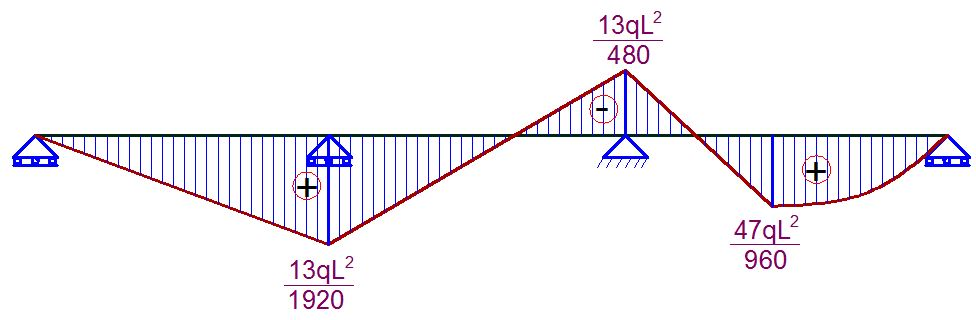Thank you visiting Structville today. Always come around…..